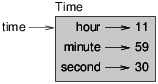Skip to main content

# 7.1: Time

As another example of a user-defined type, we’ll define a class called Time that records the time of day. The class definition looks like this:

class Time(object):
"""Represents the time of day.

attributes: hour, minute, second
"""


We can create a new Time object and assign attributes for hours, minutes, and seconds:

time = Time()
time.hour = 11
time.minute = 59
time.second = 30


The state diagram for the Time object looks like Figure 16.1.1.Figure $$\PageIndex{1}$$: Object diagram.

Exercise $$\PageIndex{1}$$

Write a function called print_time that takes a Time object and prints it in the form hour:minute:second. Hint: the format sequence '%.2d' prints an integer using at least two digits, including a leading zero if necessary.

Exercise $$\PageIndex{2}$$

Write a boolean function called is_after that takes two Time objects, t1 and t2, and returns True if t1 follows t2 chronologically and False otherwise. Challenge: don’t use an if statement.